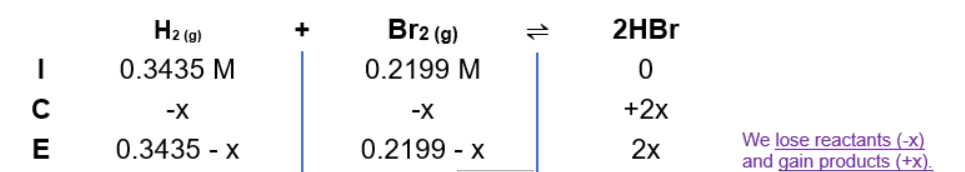# Problem: A mixture of 1.374 g of H2 and 70.31 g of Br2 is heated in a 2.00 L vessel at 700 K . These substances react as follows:H2(g) + Br2(g) 2HBr(g)At equilibrium the vessel is found to contain 0.566 g of H2.Calculate the equilibrium concentration of HBr.

###### FREE Expert Solution

Solve molarity (initial):

H2:

0.3435 M H2

Br2:

0.2199 M Br2

ICE Chart0.1415 M H2

Calculate x:

96% (1 ratings)###### Problem Details

A mixture of 1.374 g of H2 and 70.31 g of Br2 is heated in a 2.00 L vessel at 700 K . These substances react as follows:

H2(g) + Br2(g) 2HBr(g)

At equilibrium the vessel is found to contain 0.566 g of H2.

Calculate the equilibrium concentration of HBr.

Frequently Asked Questions

What scientific concept do you need to know in order to solve this problem?

Our tutors have indicated that to solve this problem you will need to apply the ICE Chart concept. You can view video lessons to learn ICE Chart. Or if you need more ICE Chart practice, you can also practice ICE Chart practice problems.

What professor is this problem relevant for?

Based on our data, we think this problem is relevant for Professor Dunning's class at TEXAS.